In Strut3D user has an option to choose the Load Type applied (Characteristic, Design). By default, application uses Characteristic Load Type option. To change the option, choose Analyze -> Calculation Parameters [Fig. 1 and Fig. 2].

Choice between Characteristic and Design Load Types entails certain consequences.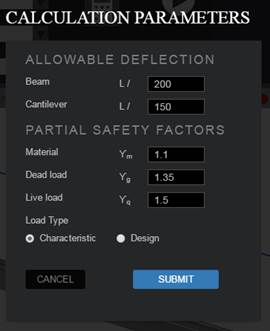Fig. 1 – Calculation parameters.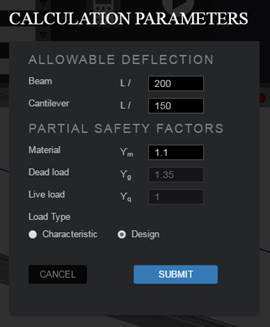Fig. 2 – Calculation parameters.

Go to application

When user decides to apply Characteristic forces, then should use correct safety factors for the Permanent Loads (Dead) and Variable (Live) in the Calculation Parameters window (Fig. 1). By default, application uses factors following „Eurocode 0”, what means 1,35 for Permanent Loads (Dead) and 1,50 for Variable (Live) Loads. At the ultimate limit state design safety factors are equal to 1,00 for each type of the load.

While defining the Characteristic load, user chooses the Nature of the load (Live/Dead) [Figure 3], then application automatically associate relevant safety factor.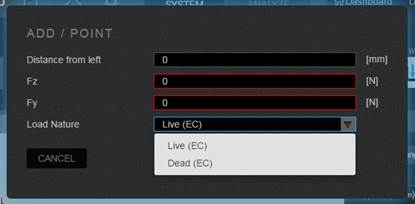Figure 3 – Point load definition dialog.
Nature of the load chosen from the list.

Load combinations are defined per the following rules:

Ultimate limit state: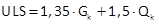Serviceability limit state: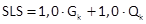Where: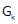– permanent action (including dead load)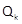– variable/live action

Go to application

When user decides to apply load as a Design, then there is no possibility to enter safety factors in the Calculation Parameters window [Figure 2]. Additionally, while applying the load there is no possibility to choose the Load Nature [Figure 4].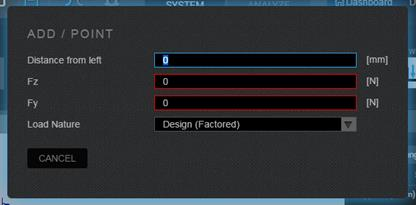In this case load combinations are defined per the following rules:

Ultimate limit state: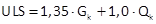Serviceability limit state: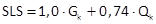Gdzie:– own weight– applied load (design)

The safety factor 0,74 for the applied loads at the serviceability limit state was evaluated from the relationship: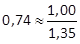. All applied loads are set into one group. To calculate deflection, the safer solution is to apply reduction factor to the applied design loads dividing them by the dead load safety factor.

Go to application